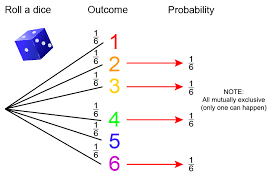## How to Calculate and Solve for Mutually Exclusive | ProbabilityThe image above represents mutually exclusive.

To compute for mutually exclusive, four essential parameters are needed and these are xA, NA, xB and NB.

The formula for calculating mutually exclusive:

P(A or B) = P(A) + P(B)

Where;

P(A or B) = Mutually Exclusive
P(A) = xANA
P(B) = xBNB

Let’s solve an example;
Find the mutually exclusive when the xA is 12, NA is 14, xB is 9 and NB is 17.

This implies that;

xA = 12
NA = 14
xB = 9
NB = 17

P(A or B) = P(A) + P(B)
P(A or B) = xANA + xBNB
P(A or B) = 1214 + 917
P(A or B) = 12(17) + 9(14)(14)(17)
P(A or B) = 204 + 126238
P(A or B) = 330238
Dividing the numerator and denominator by 2
P(A or B) = 165119
P(A or B) = 1.386

Therefore, the mutually exclusive is 1.386.